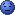CodeGuru Home VC++ / MFC / C++ .NET / C# Visual Basic VB Forums Developer.com

# Thread: pass <vector> to function

1.MemberJoin Date
May 2018
Posts
103

## pass <vector> to function

I'm making tests about <vector> and I want to pass it to function to insert some values, e.g.

Code:
```#include <iostream>
#include <vector>
#include <cstring>
using namespace std;

struct volo {
string codice;
int num;
double costo;
volo(string cd, int n, double cs): codice(cd), num(n), costo(cs) {};
};

void insert(volo* pointer, string cd_, int n_, double cs_) {
volo app(cd_, n_, cs_);
pointer.push_back(app);
}

int main() {
vector <volo> flight;

string cod;
int n;
double c;
cin >> cod >> n >> c;
insert(flight, cod, n ,c );
}```
How can I pass <vector> to function to insert values into it?
I thought <vector> was only dynamic array created by template class, so it was necessary to pass only vector nameReply With Quote

2. ## Re: pass <vector> to functionOriginally Posted by zio_mangroviaHow can I pass <vector> to function to insert values into it?
I thought <vector> was only dynamic array created by template class, so it was necessary to pass only vector name
Code:
```void someFunction(vector& <volo> flightToFillIn)
{
flightToFillIn.push_back(volo(....));
...
}
...
vector& <volo> flight; // empty vector
someFunction(flight); // now it contains something```Reply With Quote

3.MemberJoin Date
May 2018
Posts
103

## Re: pass <vector> to functionOriginally Posted by VictorN[code]
vector& <volo> flight;
I don t understand what means here & symbolReply With Quote

4. ## Re: pass <vector> to functionOriginally Posted by zio_mangroviaI don t understand what means here & symbol
It was just mistakenly copied/pasted from function definition! I am awfully sorry!It should be
Code:
```void someFunction(vector& <volo> flightToFillIn)
{
flightToFillIn.push_back(volo(....));
...
}
...
vector <volo> flight; // empty vector
someFunction(flight); // now it contains something```Reply With Quote

5.MemberJoin Date
May 2018
Posts
103

## Re: pass <vector> to function

I noted It's possible to manage <vector> even in this way:

Code:
```void printcode(volo* pp, int nn) {
for (int i=0; i<nn; ++i) {
cout << pp[i].codice << endl;
cout << pp[i].num << endl;
cout << pp[i].costo << endl;
}
}

in main()  {
vector <volo> flight;
string cod;
int N;
double c;
cin >> N;
for (int i=0; i<N; ++i) {

cin >> cod >> n >> c;
volo *app = new volo(cod, n, c);
flight.push_back(*app);
delete app;
}
printcode(&flight, N);
...
}```
Where N is number of elements.

Compiler works fine, my doubt is for &flight, It's the address of first element of <vector> as for array... but why this function call printcode(flight, N); gives error? flight is <vector> object while pp is is volo pointer !?Reply With Quote

6.Elite Member Power PosterJoin Date
Jan 2006
Location
Singapore
Posts
6,768

## Re: pass <vector> to function

This is unnecessarily convoluted:
Code:
```volo *app = new volo(cod, n, c);
flight.push_back(*app);
delete app;```
It would suffice to write:
Code:
`flight.emplace_back(cod, n, c);`
Even if you had to compile with respect to pre-C++11, it would still be simpler to write:
Code:
`flight.push_back(volo(cod, n, c));`
As for your question: yes, you get the error because -- unlike an array -- you cannot implicitly convert a vector into a pointer to its first element, hence the use of &flight.Reply With Quote

7. ## Re: pass <vector> to function

printcode() takes a pointer to volo. flight is a vector of volo. The two types are not compatible. flight gives the first element of flight and hence &flight gives the address of the first element of flight - a pointer to volo.

Note that it would be more usual to code this as flight.data() rather than &flight - both give the same.

printcode() really should be changed to

Code:
```printcode(const vector<volo>& pp)
{
for (const auto& v : pp) {
cout << v.codice << endl;
cout << v.num << endl;
cout << v.costo << endl;
}
}```

However, a more c++ way of doing this would be:

Code:
```#include <iostream>
#include <vector>
#include <cstring>
using namespace std;

struct volo {
string codice;
int num = 0;
double costo = 0.0;

volo() = default;
volo(string cd, int n, double cs) : codice(cd), num(n), costo(cs) {};
};

ostream& operator<<(ostream& os, const volo& vo)
{
return os << vo.codice << ' ' << vo.num << ' ' << vo.costo;
}

ostream& operator<<(ostream& os, const vector<volo>& vo)
{
for (const auto& v : vo)
os << v << endl;

return os;
}

istream& operator>>(istream& is, volo& vo)
{
return is >> vo.codice >> vo.num >> vo.costo;
}

int main() {
vector <volo> flight;
int N;

cin >> N;
flight.resize(N);

for (auto& f : flight) {
volo vo;

cin >> vo;
f = vo;
}

cout << flight;

//	...
}```
PS Note that this code (and the original) does not check/deal with stream errors. Also note that for codice you can't input a string using >> that contains a 'white-space' char (eg a space). Try entering the text "white space" (without the ") and see what the output is!.
Last edited by 2kaud; August 25th, 2019 at 06:23 AM. Reason: PSReply With Quote

8.Member +Join Date
Feb 2017
Posts
564

## Re: pass <vector> to functionOriginally Posted by zio_mangroviamy doubt is for &flight
Mine tooI think you should avoid &flight and flight.data().

There is no reason to use it just because you can. Save it for situations where you have no choice. Normally, if you have decided to go for an std::vector there is no reason to treat it as a mere C array unless you must.Reply With Quote

####Posting Permissions

• You may not post new threads
• You may not post replies
• You may not post attachments
• You may not edit your posts
•